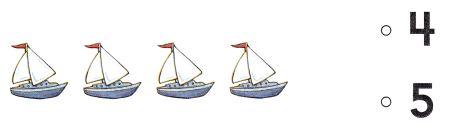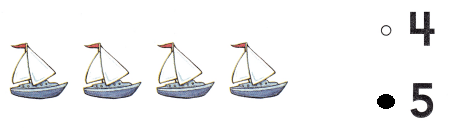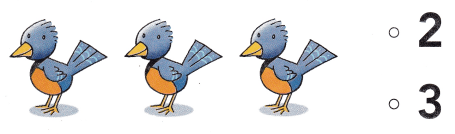# Texas Go Math Kindergarten Lesson 3.3 Answer Key Greater Than and Less Than

Refer to our Texas Go Math Kindergarten Answer Key Pdf to score good marks in the exams. Test yourself by practicing the problems from Texas Go Math Kindergarten Lesson 3.3 Answer Key Greater Than and Less Than.

## Texas Go Math Kindergarten Lesson 3.3 Answer Key Greater Than and Less Than

Explore

DIRECTIONS: Place counters as shown. Draw a line to match a counter in the top five frame to a counter below it in the bottom five frame. Tell a friend which set has a number of objects greater than the other set.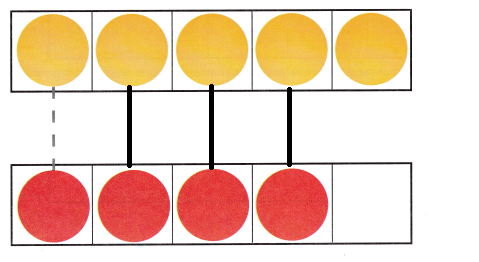Explanation:
The yellow set has more than the red counters.

Share and Show

DIRECTIONS: 1. Place counters as shown. Count and tell how many in each set. Write the numbers. Compare the sets by matching. Circle the number that is greater. 2. Place counters as shown. Count and tell how many in each set. Write the numbers. Compare the sets by matching. Circle the number that is greater.

Question 1.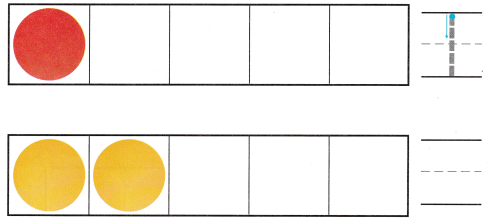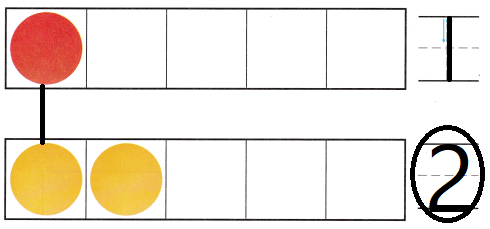Explanation:
The yellow counters are greater than the red counters
Circled the greater number

Question 2.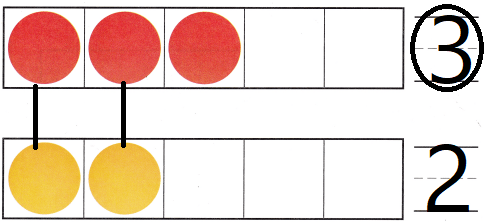Explanation:
The red circles are greater than the yellow circles
Circled the number 3

DIRECTIONS: 3. Place counters as shown. Count and tell how many in each set. Write the numbers. Compare the sets by matching. Circle the number that is less. 4. Place counters as shown. Count and tell how many in each set. Write the numbers. Compare the sets by matching. Circle the number that is less.

Question 3.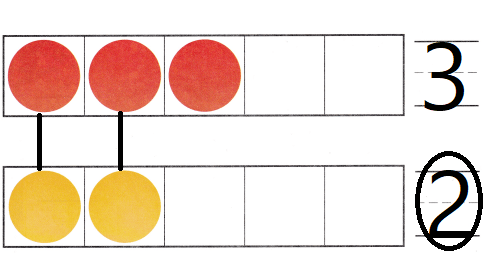Explanation:
The yellow circles are lesser than the red circles
circled the lesser number

Question 4.Explanation:
The red circles are lesser than the yellow circles
circled the lesser number

HOME ACTIVITY • Show your child two sets with o different number of objects in each set. Hove him or her use matching to compare the sets.Explanation:
The above two sets are with 0 difference
both the sets are equal

DIRECTIONS: 5. Paige found 3 shells. Pilar found 4 shells. Use counters to show how many shells each girl found. Draw the counters. Count and tell how many in each set. Circle the number that is greater. 6. Which number is greater than the number of trees? Choose the correct answer.

Problem Solving

Question 5.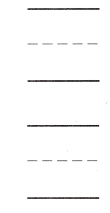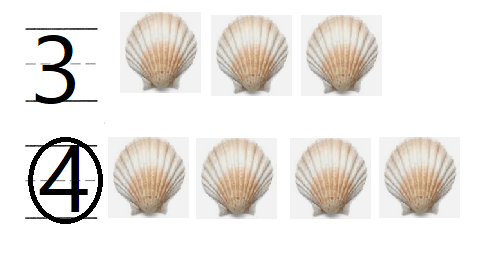Explanation:
Paige found 3 shells. Pilar found 4 shells.
Used counters to show how many shells each girl found.
Drawn the counters.
Circled the greater number

Question 6.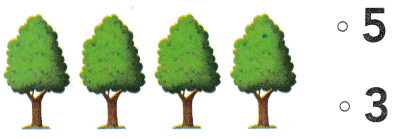Explanation:
There are 4 trees the greater number than the number of trees is 5

### Texas Go Math Kindergarten Lesson 3.3 Homework and Practice Answer Key

DIRECTIONS: 1. Count and tell how many in each set. Write the numbers. Compare the sets by matching. Circle the number that is greater. 2. Count and tell how many in each set. Write the numbers. Compare the sets by matching. Circle the number that is less.

Question 1.Explanation:
Compared and matched the counters
Circled the greater number

Question 2.Explanation:
The red circles are lesser than the yellow circles
circled the lesser number

DIRECTIONS: Choose the correct answer. 3. Which number is greater than the number of sailboats? 4. Which number is less than the number of birds?

Lesson Check

Question 3.